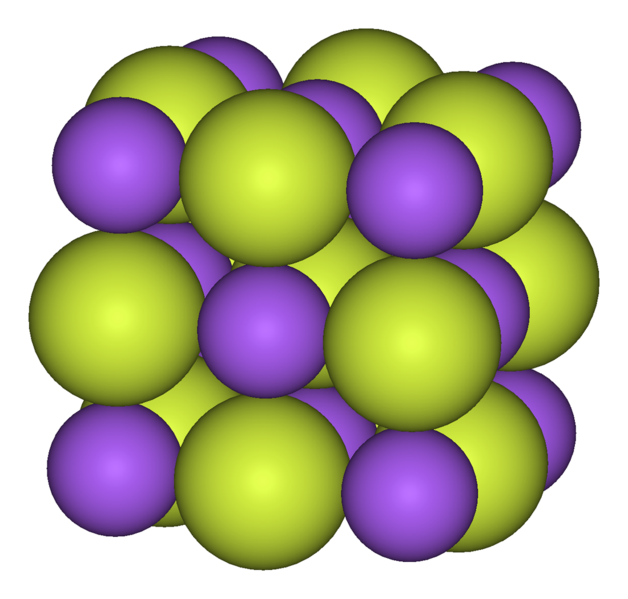Chemistry CIE iGCSE:
Bonding + Structure

Page 1

You will need a copy of the periodic table!

1. When an atom forms a positive ion it ..
• A.   gains an electron
• B.   loses an electron
• C.   gains a proton
• D.   loses a proton

16. The diagram shows the outline of part of the Periodic Table:Which two elements could form a covalent compound?

• A.   W and X
• B.   X and V
• C.   Y and W
• D.   Z and X

3. Four elements, K, L, M and N, have the following electronic configurations:

 K L M N 2,8,4 2,8,2 2,8,8 2,8,6

Which two elements react together to form a covalent compound?

• A.   K and L
• B.   L and M
• C.   M and N
• D.   N and K

Q4-5:
Sodium and fluorine react to form the compound sodium fluoride. The electronic configurations of sodium and fluorine are 2,8,1 and 2,7 respectively.4. Which of the following statements best describes the formation of sodium fluoride?
• A.   A sodium atom and a fluorine atom share a pair of electrons
• B.   A sodium atom shares a pair of electron with each of two fluorine atoms
• C.   A sodium atom transfers an electron to a fluorine atom
• D.   A fluorine atom transfers an electron to a sodium atom
5. Which statement about the melting point of sodium fluoride is true?

Sodium fluoride has a ...

• A.   .. low melting point as the forces between the ions are weak
• B.   .. low melting point as the forces between the molecules are weak
• C.   .. high melting point as the forces between the ions are strong
• D.   .. high melting point as the forces between the atoms are strong
6. Which statement best completes the description of covalent bonding?

Covalent bonding is the electrostatic attraction between ……….

• A.   positive nuclei and electrons and occurs by the sharing of electrons
• B.   positive nuclei and electrons and occurs by the transfer of electrons
• C.   positive ions and negative ions and occurs by sharing electrons
• D.   positive ions and negative ions and occurs by transfer of electrons

7. Lithium reacts with oxygen to form lithium oxide. The arrangement of the electrons in atoms of lithium and oxygen are shown in the diagram here.

Which of the following dot and cross diagram shows the arrangement of the electrons in lithium oxide?8. Which of the following molecules contains 4 shared pairs of electrons?
• A.   ammonia, NH3
• B.   hydrogen chloride, HCl
• C.   methane, CH4
• D.   water, H2O

9. The arrangement of electrons in atoms X and Y are shown in the diagram here.

X and Y form a covalent compound. What is the formula of the compound formed?• A.   XY
• B.   XY3
• C.   X3Y
• D.   X5Y

10. Two elements react to form an ionic compound with the formula MgCl2. The electronic configurations of the two elements before and after reaction are ..

 Before reaction After reaction magnesium chlorine magnesium chlorine A 2,8,2 2,8,7 2,8 2,8,8 B 2,8,2 2,8,6 2,8 2,8,8 C 2,8,2 2,8,7 2,8,1 2,8,8 D 2,8 2,8,8 2,8,2 2,8,7